# バーンダウンチャートにはどんな数字を含めるべきか？

スクラムマスターの渡部です。

スクラムでは、プロジェクトの進捗管理や問題の把握にバーンダウンチャートを使うのが良い（相性が良い）と言われています。

それは、「バーンダウンチャートに含めるべき数字とは何か？」ということです。

##### 本記事で解説する内容
• バーンダウンチャートに含めるべき数字とは何か？
##### 想定読者
• 開発で、既にバーンダウンチャートを使われている方（もしくは、使おうとしている方）

### どんな悩みを抱えていたのか？

まず、バーンダウンチャートとは、縦軸に全タスクの残り時間（ストーリーポイントなど）を置き、横軸を期間（スプリントなど）で区切ったチャートです。

スクラムの教科書では、「チームがやるべき作業を全てバックログに積むべし」との教えがありましたので、 プロジェクト内/外問わず、全ての作業をバックログにいれ、見積もりをして、完了したらその分、チャートをバーンダウンさせていました。

しかし、2〜3スプリントも完了するかどうかというときに、ふと疑問が生じました。

「元々予定してなかったタスクを後から追加して、それで着地予想は正確になるのか？」と。

### 以降の説明で使用する前提

• プロジェクトで必要な全タスクの見積もり合計：100pt
• チームが1スプリントで完了できるポイント数：10pt
• 既存サービスの運用・Bug修正・その他調査系のタスクは「プロジェクト外タスク」と呼称
• 過去3スプリントの平均ベロシティを計算し、予測線（赤色）としてチャートに表示

### パターンA：プロジェクト外タスクは一切無し

まずは、3スプリント経過時点でチャートに予測線を引いてみます。

##### 予測

チームの3スプリント平均が10ptですので、今後も10ptずつ完了されていくと仮定すると、チャートは上記のようになります。 では、時間を進めて結果を見てみましょう。

##### 結果

このパターンでは、特に問題は見られませんでした。

### パターンB：プロジェクト外タスク有り（2pt / スプリント）

##### 予測

とすると、3スプリントを終えて残り76ptですので、スプリント11にはプロジェクトは終えられるでしょうか？ 時間を進めて結果を見てみます。

##### 結果

なんと、予想から2スプリント後ろにずれてしまいました。何故でしょうか？

ここで、チャートに表示している数字の内訳を見てみたいと思います。

そうです。 チームが完了していた10ptの内、8ptしか、プロジェクトで必要なタスクを完了できていないにも関わらず、10pt完了する予測にしてしまっていたことが原因でした。

##### 予測（8ptずつ完了）

スケジュールは予想と実績で一致していますので、これであれば、より正確に予想ができそうです。

### パターンC：プロジェクト外タスク有り（5pt / スプリント）

##### 結果

スケジュールについては予想と結果が一致していますので、問題は解決できたと思います。

ですが、予測時に、実績と予測の線の傾きが異なりすぎて、直感的にイメージしにくく、他の違和感にも気づきにくいチャートになってしまっています。

これは、実績線ではプロジェクト内/外の全タスクで完了したポイントを含めているのに対し、予測線ではプロジェクト内タスクのみを含めていることが原因です。

その差を解消するためには、実績線・予測線ともに、プロジェクト内タスクのみを含める必要があります。

### パターンD：プロジェクト外タスク有り、プロジェクト内タスクのみ集計

パターンCの問題を解決するため、プロジェクト内タスクのみを含めたチャートで、予測と結果を見ていきたいと思います。

##### 結果

スケジュールも予測と結果が一致しており、予測線の傾きも直感的にイメージできるものになっているかと思います。

### 結論

##### ルール
• 下記にはプロジェクト内タスクのみ含める
• バックログ（見積もり合計）
• 実績線
• 予測線
##### 備考

ただ上記ルールにすると、プロジェクト外タスクにどれだけチームのリソースを費やしているのかがわかりませんので、次のようなグラフも併用すると良いと思います。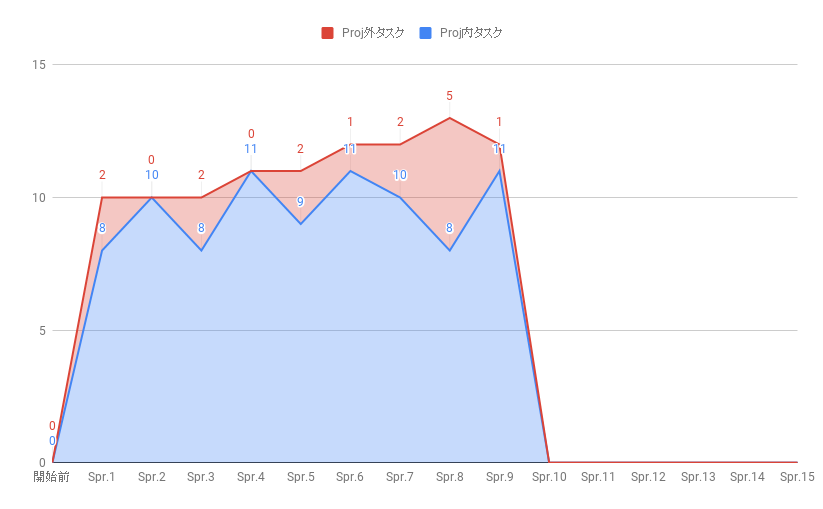### さいごに

もしくは、一緒に働きながらカイゼンしていきませんか？

ユニファでは、「世界中の家族コミュニケーションを豊かにする」ことに共にチャレンジしていく新たな仲間を積極的に募集しています！

# カルチャー・ハッカー

デザインチームの三好です。

### " ハッカーとは壁の一部が破れるはずだと常に考えている人のことである "　ジョン・ウィルスフェアー

ハッカーという言葉の意味。一般的には大いに誤解されて浸透しています。

コンピューター上で悪事を働く不正利用者のイメージがそのままハッカーとなったようですが本来は「コンピューター技術にたけて工夫ができる人」という意味になります。 ただこの場でコンピューター上のマニアックな話をするわけではなく（というよりもできない）、ハックという言葉をもっと日常的な広い意味で考えていきたいと思います。

どうやら、特に海外ではこのハックという言葉が流行ってしまっているようで「生きているだけで地球をハックしてる」「呼吸をして空気をハックしよう」など意味不明な軽いノリで使われ完全に本来の意味が崩壊していますが、真のハッカーとは『創造力を発揮して既存の常識を破壊し新たな価値観を再構築していく、挑戦を続ける人』だと私は思っています。

### マイノリティ・パワー

そのかわりに彼らの精神は自由で、余計な束縛を受けず、本当に表現したいことを強い意志で伝えることができます。

マジョリティに媚びないからこそ実現できるものがあることを知っています。

それでも一部ではありますが台湾の新たな風がヨーロッパや日本にもじわじわと浸透してきているところをみると彼らの試みは少しずつ成功してきているのだと思います。

### 企業の内側をハックする

この流れを個人的な話に置きかえてみると、自分自身を含めインハウスデザイナーというものは「企業の内側をハックする」必要があると感じています。まずはこのブログの場で誤解されがちである『デザインという言葉の意味』を浸透させることから始めていきたいと思いますが、長くなりそうなのでその話は次の機会に。

カルチャーやクリエイティブなどという言葉を使うとクリエイター職以外には無縁のように感じるかと思いますが、今は日常的にどのシーンにおいても創造性が求められる時代です。 デザイナーなどはただ単にそれを色濃く目に見える形で発信する職種であるというだけの話で、根本的には特別なものではなくどの職種にもデザイン要素は含まれていると私は思っています。

そしてそれがとても重要であるものということへの意識の底上げをすることもインハウスデザイナーの役割と考えています。

さぁ、レッツハック。# Local Binary Pattern for Local Texture Feature Extraction

By Matthew Millar R&D Scientist at ユニファ

This blog will look at how to build a Local Binary Pattern feature extractor for computer vision tasks.

### Local Binary Pattern:

What is LBP
LBP is one of many feature extractors. HOG, SIFT, SURF, FAST, DoG, etc... are all similar but do slightly different things. What makes LBP different is, its main goal is to be used for a texture descriptor on a local level. This gives a local representation of any texture of an image. This is done by comparing a pixel with the surrounding pixels. For each pixel in an image, the surrounding x number of pixels will be looked at. X can be determined and adjusted as needed. The LBP value for every pixel is calculated to its neighbors. If the center pixel is greater than or equal it's neighbor's values, then it will be set to 1, else it will be set to 0.

From the above talbe, you can see how each cell gets calculated. From this point this 2D array will be flattened to a 1D array like this:

This will giveSo 71 will be in the output image. This process will be done for every single pixel in the image.

This talbe shows how each cell is calculated:

The basic idea behind this is to calculate each value of the 1D array at each index.
The value is determined by the position of the index in the array. If the value at the index is a 1, then value calculated to bewhere i is the index position. If the value at the index is 0, then the value is set to a 0 regardless of the index position. Then you sum the results of the whole 1D array to get the center pixel value.
To get the feature vectors from this, you have to calculate a histogram first.
This will be a histogram of 256 bins as the values of the LBP can range from 0 to 255.

### Python Implementation:

OpenCV does have an LBP available, but it is meant for facial recognition and would not be appropriate for getting textures off clothing or environments. The use of the sklearn’s model can be very useful then for this project.
Let see how to implement it.

from skimage import feature
from sklearn.svm import LinearSVC
from sklearn.linear_model import LogisticRegression
import numpy as np
import cv2
import os

class LBP:
# Constructor
# Needs the radius and number of points for the outer radius
self.numPoints = numPoints
# Compute the actual lbp
def calculate_histogram(self, image, eps=1e-7):
# Create a 2D array size of the input image
lbp = feature.local_binary_pattern(image, self.numPoints,
method="uniform")
# Make feature vector
#Counts the number of time lbp prototypes appear
(hist, _) = np.histogram(lbp.ravel(),
bins= np.arange(0, self.numPoints + 3),
range=(0, self.numPoints +2))
hist = hist.astype("float")
hist /= (hist.sum() + eps)

return hist

# Create the lbp
loc_bi_pattern = LBP(12,12)
x_train = []
y_train = []

image_path = "LBPImages/"
train_path = os.path.join(image_path, "train/")
test_path = os.path.join(image_path, "test/")

for folder in os.listdir(train_path):
folder_path = os.path.join(train_path, folder)
print(folder_path)
for file in os.listdir(folder_path):
image_file = os.path.join(folder_path, file)
image = cv2.resize(image,(300,300))
gray = cv2.cvtColor(image, cv2.COLOR_BGR2GRAY)
hist = loc_bi_pattern.calculate_histogram(gray)
# Add the data to the data list
x_train.append(hist)
y_train.append(folder)


After then you can choose whichever model you want to train on. SVM I think would be best, but logistic regression or Naive Bayes could work also. It would be fun to play around with a few options to see which works best.
I trained and tested my code on images of metal and wood textures.
The results are pretty good for something so simple:

Pretty straight forward seeing we are using sklearn's implementation. All we really need to do is create the histograms to get out the feature vectors for each image. This can allow for you to then classify other images that have similar textures on them.
As you can see it works pretty well. The first “wood” image is actually metal siding, but I wanted to see how well it does one something that is very difficult to determine. This misclassification could be due to the overall image looking similar to that of wood flooring texture and not of metal textures. Even a human might have the same issue with this using a black and white photo.

### Conclusion:

The ability to extract small scale or fine grain details makes LBP a very handy tool for computer vision tasks. But, one issue is that LBP cannot capture at different scales which causes it to miss out on a global scale features. This can be overcome by using different implementations of LBP which can handle different neighborhood sizes which allows for better control over the scale. Depending on your need the use of a fixed scale or changing one might change.

https://www.pexels.com/

# AndroidXに移行してみた

こんにちは、Androidエンジニアのあいばです。

じめじめと嫌な天気が続いていますが、いかがお過ごしでしょうか。

## AndroidXについて

AndroidX の概要  |  Android Developers

## 今回のゴール

AndroidX移行したプロジェクトをCircleCIでビルド＆配信できること

## 早速やってみる

### Auto migration

まずはこちらを参考にauto migrationを実行しました。

Migrating to AndroidX  |  Android Developers

Android Studioのメニューバーから [Refactor] > [Migrate to AndroidX] を実行するとgradle.propertiesに次の2つのフラグが追加され、Android Studio のビルドシステムにより依存関係も自動的に移行されます。

android.useAndroidX=true
android.enableJetifier=true


#### 追加で必要だった対応

• DataBindingについて次のエラーが発生
ERROR: Data Binding annotation processor version needs to match the Android Gradle Plugin version.
You can remove the kapt dependency androidx.databinding:databinding-compiler:1.0.0 and Android Gradle
Plugin will inject the right version.

次の行を削除する事で解決しました。

    kapt 'androidx.databinding:databinding-compiler:1.0.0'


Android Studio Canary8から不要になっていたそうです。
DataBindingのkaptを書かなくても良くなった - Kenji Abe - Medium

• 次のようにexcludeなどでサポートライブラリを指定している箇所は自動で移行されないため必要に応じて削除、修正が必要です。
    implementation("com.squareup.picasso:picasso:\$picassoVersion") {
exclude group: "com.android.support", module: "exifinterface"
}

• 自動で移行されたライブラリはバージョン1.0.0と指定されているのでその時の最新バージョンに更新します。
##### src
• importは正しく修正されていますが、コード内でも完全修飾で参照してしまっているので修正します。
• RecyclerView.ItemDecoration.onDraw(Canvas, RecyclerView, RecyclerView.State)など引数がNonNullに変更されたoverrideメソッドでエラーになるので修正が必要です。

#### テスト

ここまでの作業を終えてテストを実行したところ…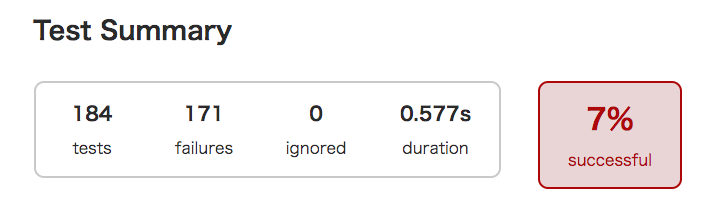Robolectricを利用しているテストが初期化で失敗していました。 Robolectricのバージョンが3.xと古かったため最新にして再びテスト実行します。

android {
testOptions {
unitTests.all {
maxHeapSize = "1g"
}
}
}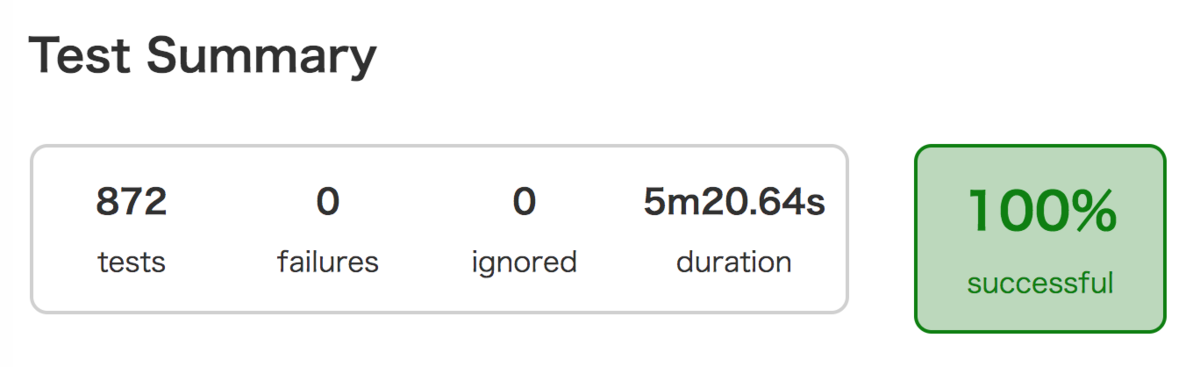#### CircleCIでビルド

テスト実行時に先ほど倒したはずのOutOfMemoryErrorが発生してます。
どうやらCircleCI 上のコンテナで使用できるメモリが不足しているようだったので、CircleCIのプランを見直しresource_classオプションを利用することにしました。

Configuring CircleCI - CircleCI

# Hadoop from Start to Finish on Windows10

By Matthew Millar R&D Scientist at ユニファ

This blog will be looking at how to set up and start a Hadoop server on windows as well as give some explanation as to what it is used for.

Hadoop is a set of tools that can be used for easy processing and analyzing Big Data for a company and research. Hadoop gives you tools to manage, query, and share large amounts of data with people who are dispersed over a large geographical location. This means that teams in Tokyo can easily work with teams in New York, well not accounting for sleeping preferences. Hadoop gives a huge advantage over a traditional storage system, not only in the total amount of storage possible, but in flexibility, scalability, and speed of access to this data.

### Modules

Hadoop is split up into 4 distinctive modules. Each module performs a certain task that is needed for the distributed system to function properly. A distributed system is a computer system that has its components separated over a network of different computers. This can be both data and processing power. The actions of the computers are coordinated by messages that are passed back and forth between each other. These systems are complex to set up and maintain but offer a very powerful network to process large amounts of data or run very expensive jobs quickly and efficiently.

The first module is the Distributed Filesystem. The HDFS allows files to be stored, processed, shared and managed across a set of connected storage devices. HDFS is not like a regular operating file system and normally can be accessed by any supported OS which gives a great deal of freedom.

The second module is MapReduce. There are two main functions that this module performs. Mapping is the act of reading in the data (or gathering it form each node). Mapping then puts all this data into a format that can be used for analysis. Reduce can be considered the place where all the logic is performed on the collected data. In other words, Mapping gets the data, Reducing analyzes it.

Hadoop common is the third module. This module consists of a set of Java tools that each OS needs to access and read the data that is stored in the HDFS.

The final module is YARN which is the system management that manages the storing of the data and running of task/analysis of the data.

### Little More Detail:

What is a namenode? A namenode stores all the metadata of all the files in the HDFS. This includes permissions, names, and block locations. These blocks can be mapped to each datanode. The namenode is also responsible for managing the datanode, i.e. where it is saved, which blocks are on which node, etc…
A datanode, aka a slave node, is the node that actually stores and retrieves blocks of information requested by the namenode.

### Installation:

Now with the background out of the way lets try to install the system on Windows 10.

Step 2: Make its own folder in the C drive to keep things tidy and make sure that it is easy to find.
NOTE DO NOT PUT ANY SPACES AS IT CAN CAUSE SOME VARIABLES TO IMPROPERLY EXPAND.

Step 3: Unpack the tar.gz file (I suggest 7 zip as it works on windows and is free)

Step 4: To run it on Windows, you need a windows compatible binary from this repo

Step 5: Create a folder called data and, in this folder, create two others called datanode and namenode. The datanode will hold all the data that is assigned to it. The namenode is the master node which holds the metadata for the datanode (i.e. which data node the 64mb blocks is located on)

Step 6: Set up Hadoop Environment variables like so:

HADOOP_HOME=”C:\BigData\hadoop-2.9.1\bin”


Step 7: Editing several configuration files.
First up is the:

set HADOOP_PREFIX=%HADOOP_HOME%


Next, let's look at:

<configuration>
<property>
<name>fs.default.name</name>
<value>hdfs://0.0.0.0:19000</value>
</property>
</configuration>


<configuration>
<property>
<name>dfs.replication</name>
<value>1</value>
</property>
<property>
<name>dfs.namenode.name.dir</name>
</property>
<property>
<name>dfs.datanode.data.dir</name>
</property>
</configuration>


And now the:

<configuration>
<property>
<name>mapreduce.job.user.name</name>
</property>
<property>
<name>mapreduce.framework.name</name>
<value>yarn</value>
</property>
<property>
<name>yarn.apps.stagingDir</name>
</property>
<property>
<value>local</value>
</property>
</configuration>


On the first time you start up you need to run I a cmd write:
Now cd into your sbin folder and type

start-all.cmd

This will open up 4 other screens like this

Their names are; namenode, datanode, nodemanager, and resourcemanager.
And now we can look at Hadoop in a browser
The resource manager is
http://localhost:8088/cluster/cluster
This is what you should be greeted with

Here are the links for the; node manager, datanode, and a manager for Hadoop.
http://localhost:8042/node
http://localhost:50070/dfshealth.html#tab-overview
http://localhost:50075/datanode.html

Now we can finally start to work or using Hadoop after all this set and configuration.
Open a cmd
To insert data into the HDFS use the following

fs -mkdir /user/input
fs -put /home/file.txt /user/input
fs -ls /user/input 

To retrieve the Data from HDFS use the following

fs -cat /user/output/outfile
fs -get /user/output/ /home/hadoop_tp/ 

And finally shut down the system.

stop-dfs.sh

And that’s it, we managed to install and use Hadoop. Now this is a very simple way of doing it and there may be better approaches like using Dockers, or commercial versions which are much easier to use and setup, but learning how to set it up and run it from scratch is a good experience.

### Conclusion

We learned that it was very complex to set up and configure all of Hadoop. But with all the power it can bring to Big Data analysis as well as large data sets that are used for AI training and testing, Hadoop can be a very powerful tool in any researcher, data scientist, and business intelligence analyst.
A potential use of Hadoop form image analysis where you have images that are stored in different sources or if you want to use a standard set of images, but the number of images is too large to store locally in a traditional storage solution. Using Hadoop, one can establish a feeding reducer that can then be used in a data generator method in a Keras model. The potentially one can have an endless stream of data from an extremely large dataset, thus giving almost unlimited data. This approach can also be used to get numerical data that is stored on a Hadoop system. Now you do not have to download the data directly just use Hadoop to query and do preprocessing of the data before you feed it into your model. This can save time and energy when working with distributed systems.

# SIFT for Feature Extraction in a Image in Python

### Feature Extraction SIFT (Scale Invariant Feature Transform)

Why am I making this post?
Well, I basically needed to make my own SIFT algorithm as there is no free one in OpenCV anymore, well at least 3.0+.
For computer vision, one of the most basic ideas is to extract information from an image. This is feature extraction. There are different levels of features mainly global and local features. This blog will look at SIFT which is a local feature extractor. This is done by finding key points or areas of great change then adds quantitative information or descriptors that can then be used in a more complex task like object detection. Ideally, these key points should be able to be uniquely identified in various images regardless of transformations or changes in the image.

Why Python?
Yes, it's not the best in speed for this, and after running the code it takes a hot minute for it to do the feature extraction. But, I can easily use it in any computer vision project that I have now and it plugs and play no problem.

### How does SIFT work?

First, you give SIFT a picture to work with, we will be using an image I took of a dog from when I went dog sledding in Finland.
Step1: Double the size of your image both using bilinear interpolation.
Step 2: Blur the image using Gaussian Convolution.
Step 3: Preform more convolutions using Standard Deviation.
Step 4: Downsample each image.
Step 5: Restart the convolution again.
Continue this until the image is too small to perform these steps anymore.
This is called a scale-space which will help simulate many different scales that an image can come in (i.e. from small to larger and everything in between).

After the convolution, we will have to get the Laplacian for each scale space. This gives a grey scale value for each element in the image. The max values for the Laplacian will then be our key points. The max pixel will be a pixel whose value is larger than all its surrounding other pixels. This can be extended to several pixels or even a larger area depending on your needs. We can refine the key point results in the scale space by using a Quadratic Taylor expansion. Along with this, the identification of key points that lie on the edge of an object should also be removed as they are poor key points as they are not unique to translations of an image and are parallel to the edge direction. The point of keypoint extractions is not to find an edge of an object, but rather to find unique features of the image which may or may not lie on the edge of a target. That is the difference between edge detection and key point detection.

Finally, SIFT will give a reference orientation to each key point. Then the gradient of the image will be calculated by finite differences. Then smooth the gradients of the image using box blurs. This will allow for the remaining points that exceed a certain value/threshold to be kept. Another key point will be discarded.

After all of that, we have a list of final key points that we can create the descriptors for. This is done by creating a histogram of the gradient directions for each key point. This will not just make one histogram as it will make several around in a circle of the pixel where the histogram corresponds to the center pixel. Each gradient is a circle shape (rather than a box as used previously), and any key point that cannot create a full circle will be discarded.

So, after all of this, we now are left with a set of key points that are local features that can help us identify unique objects in images. This can then be used on its own for simple computer vision tasks like object identification, image slicing, and binding, or even image similarity for search engines. So, SIFT can be very useful if you know how and why it works.

### Code for SIFT

Here is the pseudo code for getting SIFT for key point detection.

Sift Code
Find_Key_Points(image):
Gaussian Smoothing (image)
Downsample the image
Make Gaussians Pyramids
Create Downsample Gaussians Pyramids
For each octave:
Start Extrema detection.

for each image sample at each scale:
find the orientation

Calculate each keypoints orientation
Calculate each keypoints descriptor


As you can see the key points found in this image is not perfect, but the implementation of SIFT is not very easy. Also, this image has a lot of noise in it so it may look like the algorithm did not work, but it is working fine, but the changes are on such a small level that it is difficult to even see it.

And for those who want to see here is the whole (all be it abridged) code

import numpy as np
from scipy import signal
from scipy import misc
from scipy import ndimage
from scipy.stats import multivariate_normal
from numpy.linalg import norm
import numpy.linalg

def sift_generate_keypoints(imagename, threshold):
s = 3
k = 2 ** (1.0 / s)

# Standard deviations for Gaussian smoothing
vector_1 = np.array([1.3, 1.6, 1.6 * k, 1.6 * (k ** 2), 1.6 * (k ** 3), 1.6 * (k ** 4)])
vector_2 = np.array([1.6 * (k ** 2), 1.6 * (k ** 3), 1.6 * (k ** 4), 1.6 * (k ** 5), 1.6 * (k ** 6), 1.6 * (k ** 7)])
...
vector_total = np.array([1.6, 1.6 * k, 1.6 * (k ** 2), 1.6 * (k ** 3), 1.6 * (k ** 4), 1.6 * (k ** 5), 1.6 * (k ** 6), 1.6 * (k ** 7), 1.6 * (k ** 8), 1.6 * (k ** 9), 1.6 * (k ** 10), 1.6 * (k ** 11)])

# Downsampling images
doubled = misc.imresize(original, 200, 'bilinear').astype(int)
normal = misc.imresize(doubled, 50, 'bilinear').astype(int)
halved = misc.imresize(normal, 50, 'bilinear').astype(int)
quartered = misc.imresize(halved, 50, 'bilinear').astype(int)

# Initialize Gaussian pyramids
pyramid_l_1 = np.zeros((doubled.shape, doubled.shape, 6))
pyramid_l_2 = np.zeros((normal.shape, normal.shape, 6))
pyramid_l_3 = np.zeros((halved.shape, halved.shape, 6))
pyramid_l_4 = np.zeros((quartered.shape, quartered.shape, 6))

# Gaussian pyramids
for i in range(0, 6):
pyramid_l_1[:,:,i] = ndimage.filters.gaussian_filter(doubled, kvec1[i])
...
pyramid_l_4[:,:,i] = misc.imresize(ndimage.filters.gaussian_filter(doubled, kvec4[i]), 1.0 / 8.0, 'bilinear')

# Difference-of-Gaussians (DoG) pyramids
dog_pyrd_l_1 = np.zeros((doubled.shape, doubled.shape, 5))
...
dog_pyrd_l_4 = np.zeros((quartered.shape, quartered.shape, 5))

# Construct DoG pyramids
for i in range(0, 5):
dog_pyrd_l_1[:,:,i] = pyrlvl1[:,:,i+1] - pyrlvl1[:,:,i]
...
dog_pyrd_l_4[:,:,i] = pyrlvl4[:,:,i+1] - pyrlvl4[:,:,i]

# extrema locations pyramids
extrem_loc_l_1 = np.zeros((doubled.shape, doubled.shape, 3))
...
extrem_loc_l_4 = np.zeros((quartered.shape, quartered.shape, 3))

for i in range(1, 4):
for j in range(80, doubled.shape - 80):
for k in range(80, doubled.shape - 80):
if np.absolute(dog_pyrd_l_1[j, k, i]) < threshold:
continue

maxbool = (dog_pyrd_l_1[j, k, i] > 0)
minbool = (dog_pyrd_l_1[j, k, i] < 0)

for di in range(-1, 2):
for dj in range(-1, 2):
for dk in range(-1, 2):
if di == 0 and dj == 0 and dk == 0:
continue
maxbool = maxbool and (dog_pyrd_l_1[j, k, i] > dog_pyrd_l_1[j + dj, k + dk, i + di])
minbool = minbool and (dog_pyrd_l_1[j, k, i] < dog_pyrd_l_1[j + dj, k + dk, i + di])
if not maxbool and not minbool:
break

if not maxbool and not minbool:
break

if not maxbool and not minbool:
break
if maxbool or minbool:
dx = (dog_pyrd_l_1[j, k+1, i] - dog_pyrd_l_1[j, k-1, i]) * 0.5 / 255
dy = (dog_pyrd_l_1[j+1, k, i] - dog_pyrd_l_1[j-1, k, i]) * 0.5 / 255
ds = (dog_pyrd_l_1[j, k, i+1] - dog_pyrd_l_1[j, k, i-1]) * 0.5 / 255
dxx = (dog_pyrd_l_1[j, k+1, i] + dog_pyrd_l_1[j, k-1, i] - 2 * dog_pyrd_l_1[j, k, i]) * 1.0 / 255
dyy = (dog_pyrd_l_1[j+1, k, i] + dog_pyrd_l_1[j-1, k, i] - 2 * dog_pyrd_l_1[j, k, i]) * 1.0 / 255
dss = (dog_pyrd_l_1[j, k, i+1] + dog_pyrd_l_1[j, k, i-1] - 2 * dog_pyrd_l_1[j, k, i]) * 1.0 / 255
dxy = (dog_pyrd_l_1[j+1, k+1, i] - dog_pyrd_l_1[j+1, k-1, i] - dog_pyrd_l_1[j-1, k+1, i] + dog_pyrd_l_1[j-1, k-1, i]) * 0.25 / 255
dxs = (dog_pyrd_l_1[j, k+1, i+1] - dog_pyrd_l_1[j, k-1, i+1] - dog_pyrd_l_1[j, k+1, i-1] + dog_pyrd_l_1[j, k-1, i-1]) * 0.25 / 255
dys = (dog_pyrd_l_1[j+1, k, i+1] - dog_pyrd_l_1[j-1, k, i+1] - dog_pyrd_l_1[j+1, k, i-1] + dog_pyrd_l_1[j-1, k, i-1]) * 0.25 / 255

dD = np.matrix([[dx], [dy], [ds]])
H = np.matrix([[dxx, dxy, dxs], [dxy, dyy, dys], [dxs, dys, dss]])
x_hat = numpy.linalg.lstsq(H, dD)
D_x_hat = dog_pyrd_l_1[j, k, i] + 0.5 * np.dot(dD.transpose(), x_hat)

r = 10.0
if ((((dxx + dyy) ** 2) * r) < (dxx * dyy - (dxy ** 2)) * (((r + 1) ** 2))) and (np.absolute(x_hat) < 0.5) and (np.absolute(x_hat) < 0.5) and (np.absolute(x_hat) < 0.5) and (np.absolute(D_x_hat) > 0.03):
extrem_loc_l_1[j, k, i - 1] = 1

#........
# Repeat for each octave

...

ori_pyramid_l_1 = np.zeros((doubled.shape, doubled.shape, 3))
...
ori_pyramid_l_4 = np.zeros((quartered.shape, quartered.shape, 3))

for i in range(0, 3):
for j in range(1, doubled.shape - 1):
for k in range(1, doubled.shape - 1):
grad_mag_ori_l_1[j, k, i] = ( ((doubled[j+1, k] - doubled[j-1, k]) ** 2) + ((doubled[j, k+1] - doubled[j, k-1]) ** 2) ) ** 0.5
ori_pyramid_l_1[j, k, i] = (36 / (2 * np.pi)) * (np.pi + np.arctan2((doubled[j, k+1] - doubled[j, k-1]), (doubled[j+1, k] - doubled[j-1, k])))

# Repeat for each orientation pyramid

extr_sum = int(np.sum(extrem_loc_l_1) + np.sum(extrem_loc_l_2) + np.sum(extrem_loc_l_3) + np.sum(extrem_loc_l_4))
keypoints = np.zeros((extr_sum, 4))

#Key point calculation
count = 0

for i in range(0, 3):
for j in range(80, doubled.shape - 80):
for k in range(80, doubled.shape - 80):
if extrem_loc_l_1[j, k, i] == 1:
gaussian_window = multivariate_normal(mean=[j, k], cov=((1.5 * vector_total[i]) ** 2))
two_sd = np.floor(2 * 1.5 * vector_total[i])
orient_hist = np.zeros([36,1])
for x in range(int(-1 * two_sd * 2), int(two_sd * 2) + 1):
ylim = int((((two_sd * 2) ** 2) - (np.absolute(x) ** 2)) ** 0.5)
for y in range(-1 * ylim, ylim + 1):
if j + x < 0 or j + x > doubled.shape - 1 or k + y < 0 or k + y > doubled.shape - 1:
continue
weight = grad_mag_ori_l_1[j + x, k + y, i] * gaussian_window.pdf([j + x, k + y])
bin_idx = np.clip(np.floor(ori_pyramid_l_1[j + x, k + y, i]), 0, 35)
orient_hist[int(np.floor(bin_idx))] += weight

maxval = np.amax(orient_hist)
maxidx = np.argmax(orient_hist)
keypoints[count, :] = np.array([int(j * 0.5), int(k * 0.5), vector_total[i], maxidx])
count += 1
orient_hist[maxidx] = 0
newmaxval = np.amax(orient_hist)
while newmaxval > 0.8 * maxval:
newmaxidx = np.argmax(orient_hist)
np.append(keypoints, np.array([[int(j * 0.5), int(k * 0.5), vector_total[i], newmaxidx]]), axis=0)
orient_hist[newmaxidx] = 0
newmaxval = np.amax(orient_hist)
# Repeat for each octave
# Create descriptors

magnit_py = np.zeros((normal.shape, normal.shape, 12))
orient_py = np.zeros((normal.shape, normal.shape, 12))

for i in range(0, 3):
magnit_py[:, :, i] = misc.imresize(grad_mag_ori_l_1[:, :, i], (normal.shape, normal.shape), "bilinear").astype(float)
magnit_py[:, :, i] = (magmax / np.amax(magnit_py[:, :, i])) * magnit_py[:, :, i]
orient_py[:, :, i] = misc.imresize(ori_pyramid_l_1[:, :, i], (normal.shape, normal.shape), "bilinear").astype(int)
orient_py[:, :, i] = ((36.0 / np.amax(orient_py[:, :, i])) * orient_py[:, :, i]).astype(int)

for i in range(0, 3):
magnit_py[:, :, i+3] = (magpyrlvl2[:, :, i]).astype(float)
orient_py[:, :, i+3] = (oripyrlvl2[:, :, i]).astype(int)

for i in range(0, 3):
magnit_py[:, :, i+6] = misc.imresize(magpyrlvl3[:, :, i], (normal.shape, normal.shape), "bilinear").astype(int)
orient_py[:, :, i+6] = misc.imresize(oripyrlvl3[:, :, i], (normal.shape, normal.shape), "bilinear").astype(int)

for i in range(0, 3):
magnit_py[:, :, i+9] = misc.imresize(grad_mag_ori_l_4[:, :, i], (normal.shape, normal.shape), "bilinear").astype(int)
orient_py[:, :, i+9] = misc.imresize(ori_pyramid_l_4[:, :, i], (normal.shape, normal.shape), "bilinear").astype(int)

descriptors = np.zeros([keypoints.shape, 128])

for i in range(0, keypoints.shape):
for x in range(-8, 8):
for y in range(-8, 8):
theta = 10 * keypoints[i,3] * np.pi / 180.0
xrot = np.round((np.cos(theta) * x) - (np.sin(theta) * y))
yrot = np.round((np.sin(theta) * x) + (np.cos(theta) * y))
scale_idx = np.argwhere(vector_total == keypoints[i,2])
x0 = keypoints[i,0]
y0 = keypoints[i,1]
gaussian_window = multivariate_normal(mean=[x0,y0], cov=8)
weight = magnit_py[int(x0 + xrot), int(y0 + yrot), scale_idx] * gaussian_window.pdf([x0 + xrot, y0 + yrot])
angle = orient_py[int(x0 + xrot), int(y0 + yrot), scale_idx] - keypoints[i,3]
if angle < 0:
angle = 36 + angle

bin_idx = np.clip(np.floor((8.0 / 36) * angle), 0, 7).astype(int)
descriptors[i, 32 * int((x + 8)/4) + 8 * int((y + 8)/4) + bin_idx] += weight

descriptors[i, :] = descriptors[i, :] / norm(descriptors[i, :])
descriptors[i, :] = np.clip(descriptors[i, :], 0, 0.2)
descriptors[i, :] = descriptors[i, :] / norm(descriptors[i, :])

return [keypoints, descriptors]


Now for a more real-world example. Getting data from the Market1501 data set that holds several images of people for different tasks. By running one of the images through the key point extractor, it allows for you to find the local features that are unique to the image itself.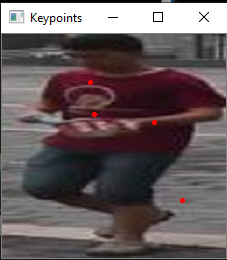PersonKeypointFrom the above picture you can see that a few of the key points are generated that look great, and one that is over on the street which is what you do not want. This is not the SIFT extractors fault it is looking over the whole image and not just the person. If you want a better result for a person's individual key points without the background mess, I would suggest using segmentation to create a mask and then use the results in the SIFT extractor. That way it will limit what is being looked at and will give better local features for each person.

### Conclusion

This implementation is not the state of the art method for getting key points and is very susceptible to blurry or poor image quality, as well as background noise and noise in the image that you cannot detect yourself. This took a long while to implement and the results are not that great compared to the time it took to create this. In my opinion, I would look into other means of extracting local features like the segmentation of the image.

### Reference

Otero, I. R., & Delbracio, M. (2014). Anatomy of the SIFT Method. Image Processing On Line,4, 370-396. doi:10.5201/ipol.2014.82

Market data set retrived from here:
https://jingdongwang2017.github.io/Projects/ReID/Datasets/Market-1501.html

# ユニファのエンジニア採用に興味がある人をベイズ推定で見極める

#### はじめに

こんにちわ、研究開発部の島田です。

さて、会社としての学会参加の目的はいくつかあるわけですが、その中の一つとして”採用”があります。ですので、ブース対応するユニファ社員の関心事の一つとして、この人は「採用に興味があるのか、無いのか」になります。

ということで、前置きが長くなりましたが今回の記事は、学会のブースに来た人が採用に興味があるか否かを数値化してみたいと思います。

※ただし、本記事で計算に使う各パラメータは全て架空のパラメータとします。

#### ベイズ推定

さて、目的の数値化のために、今回はベイズ推定を使いたいと思います。

ベイズ推定は、一般的には下記のように説明されます。

ベイズ確率の考え方に基づき、観測事象（観測された事実）から、推定したい事柄（それの起因である原因事象）を、確率的な意味で推論することを指す。

ベイズ統計の特徴（強み）は以下があげられます。

• 事前知識を取り入れることで、少ないデータでもある程度の精度で推測可能。

• 入力情報によって瞬時にそして自動的に推測を更新する

• 逐次合理性が成り立つ

#### ベイズ推定の流れ

はじめに今回のベイズ推定の処理の流れについて見ていきます。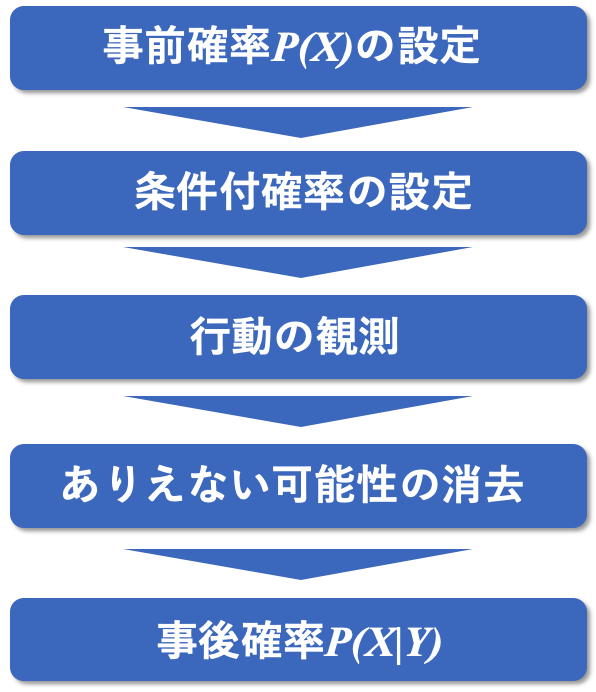#### 事前確率の設定#### 条件付き確率の設定

• 「採用に興味がある かつ 声をかける()」割合が80%(0.8)

• 「採用に興味がある かつ 声をかけない()」割合が20%(0.2)

• 「採用に興味がない かつ 声をかける()」割合が40%(0.4)

• 「採用に興味がない かつ 声をかけない()」割合が60%(0.6)

#### 行動の観測、ありえない可能性の消去

これは一人の行動を実際に観測したことになり、「声かけしない」という可能性が消えて、確率が変化しました。

つまり、この実際の観測により、世界は以下の二つに限定されました。

1. 「採用に興味ある＆声かける」P(A and 声かけ)

2. 「採用に興味ない＆声かける」P(B and 声かけ)

それぞれの確率を計算します。#### 事後確率を求める

では、いよいよ本題である「声かけをしてきた人で採用に興味がある確率」を求めます。

そこで、それぞれの確率の組を正規化（足して1になるようにする処理のこと）を行い、事後確率を求めます。この結果から、声をかけていただいた人が「採用に興味がある」確率は、約18%であると推定できました。

このようにデータが与えられる度に値が更新されていくのもベイズ推定の一つの特徴でもあります。

#### おわりに

ベイズ推定を簡単に応用したケースを紹介してみましたが、いかがでしたでしょうか。

ベイズ推定は実際のビジネスシーンにおいてもかなり幅広く応用できる手法ですので、今後も色々と試してみたいと思います。

また、冒頭でもお伝えした通り、今回のブース出展では大変多くの方に応援のメッセージを頂きました！皆さんの期待を裏切らないためにも日々精進していきたいと思います。